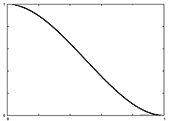# Geometric Distance Algorithm for Finding Initial Vertex WeightsThis initial weight guessing algorithm uses mesh connectivity and geometric distance rather than Euclidean distance to decide which joints should influence a given vertex. This prevents a joint from influencing a vertex that, although very close in Euclidean distance, is not near to the joint in terms of the mesh. A classic example of this is in the knees of a biped character. The vertices at the inside of the knees may be very close to each other but you would never want a left knee joint to influence a right knee vertex, or vice versa.

The geometric distances between vertices is precomputed using Dijkstra's algorithm using each vertex as the source. This is an NxN matrix, for a mesh of N vertices. This is one way to compute All Pairs Shortest Paths for a graph, or in my case, a mesh.

So, you might ask, how do you compute distance from a vertex to a joint? The answer, cut the mesh with the joint coordinate plane and the store the "ring" of vertices in the mesh that approximate the plane-mesh intersections. This ring of vertices are at distance 0 from the joint, then use the precomputed All Pairs Shortest Paths matrix for the rest.

Below is a psuedocode outline of the algorithm:

```/* Cut the mesh by each joint and get the ring of vertices. */
for each joint j in skeleton,
Cut the mesh by the plane defined by j's coordinate system
Store list of n vertices in the mesh that best approximate
the n plane-mesh intersections

/* Find initial influence set and weights for each vertex */
for each vertex v in mesh,
Find the geometric distance d to each joint by finding the nearest vertex
in each plane-mesh approximation list

do,
if the current closest joint dj = min( D ) < Threshold,
Add joint j to v's influence set with weight = dj
D = D - dj

until we have selected the maximum number of influences

Maximum-Distance = min( D )

for each influence j,
weightj = F( weightj / Maximum-Distance )

```

For the weight normalization function F I use a Cubic Hermite Spline. I tried a linear falloff function as well but this one produced qualitatively better results.Luke Tokheim, <ltokheim@cs.wisc.edu>, May 13, 2003